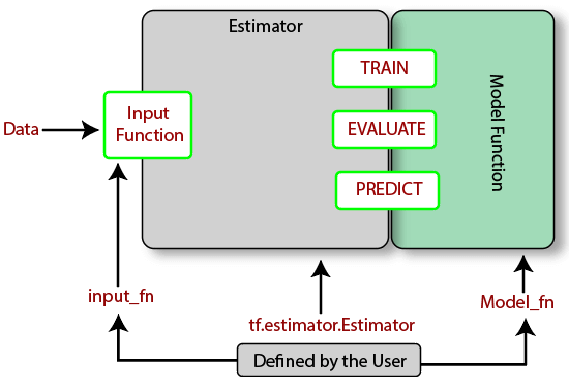What do you understand by TensorFlow estimators?

Estimators is a high-level API which reduces much of the code you previously needed to write while training a TensorFlow model. Estimators are very flexible, and it allows to override the default behavior if there is any specific requirement for the model.

There are two possible ways of building a model using Estimators:

Pre-made Estimator: These are predefined estimators. These are created to generate a specific type of model, e.g., DNNClassifier, which is a pre-made estimator.

Estimator (base class): It provides complete control of how a model should be created by using a model_fn function. These functions are consumed by the class tf.estimator.Estimator. The functions return an initialized estimator, upon which we can call .train.eval, and .predict.

A schematic of Estimator can be seen as: